Math resources Number and quantity

# Number and quantity

Here you will find all the number and quantity resources you need. Like all our math topic guides, we've included step-by-step guides, detailed examples and practice questions!

Filter by:

Arithmetic

Types of numbers

Multiplication and division

Factors and multiples

Fractions operations

Exponents

Properties of equality

Rounding numbers

Decimals

Fractions

Show all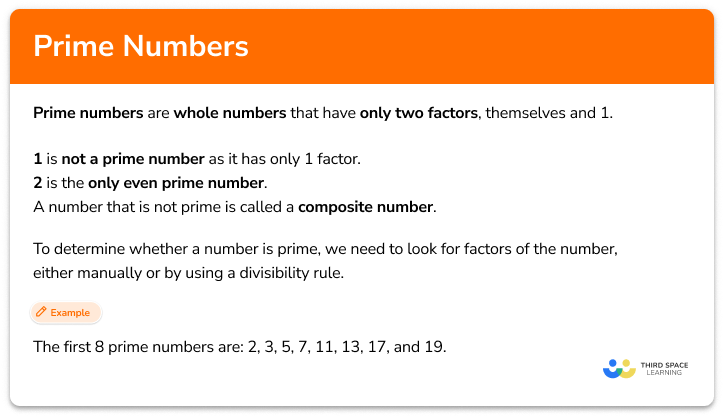Prime numbers

Prime numbers value step-by-step topic guide, detailed examples, practice questions, teaching tips and FAQs.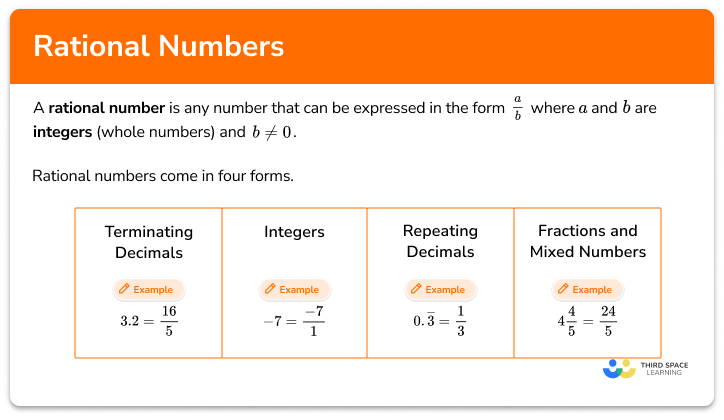Rational numbers

Rational numbers step-by-step topic guide, detailed examples, practice questions, teaching tips and FAQs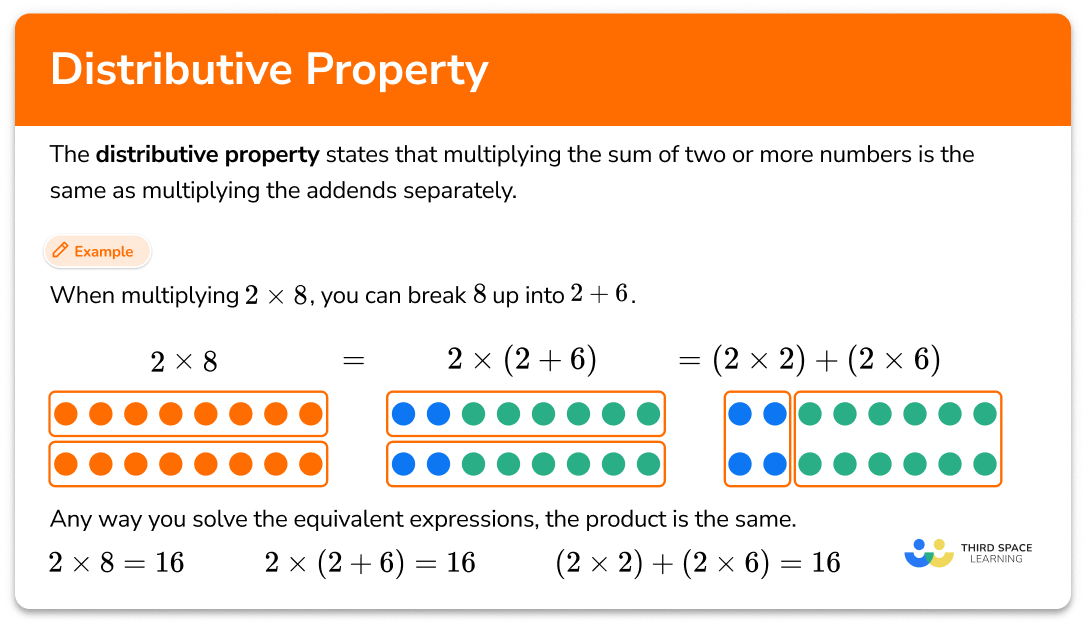Distributive property

Distributive property step-by-step topic guide, detailed examples, practice questions, teaching tips and FAQs.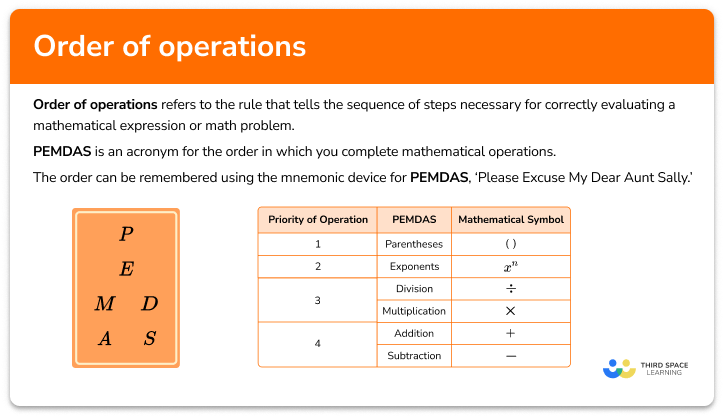Order of operations

Order of operations step-by-step topic guide, detailed examples, practice questions, teaching tips and FAQs.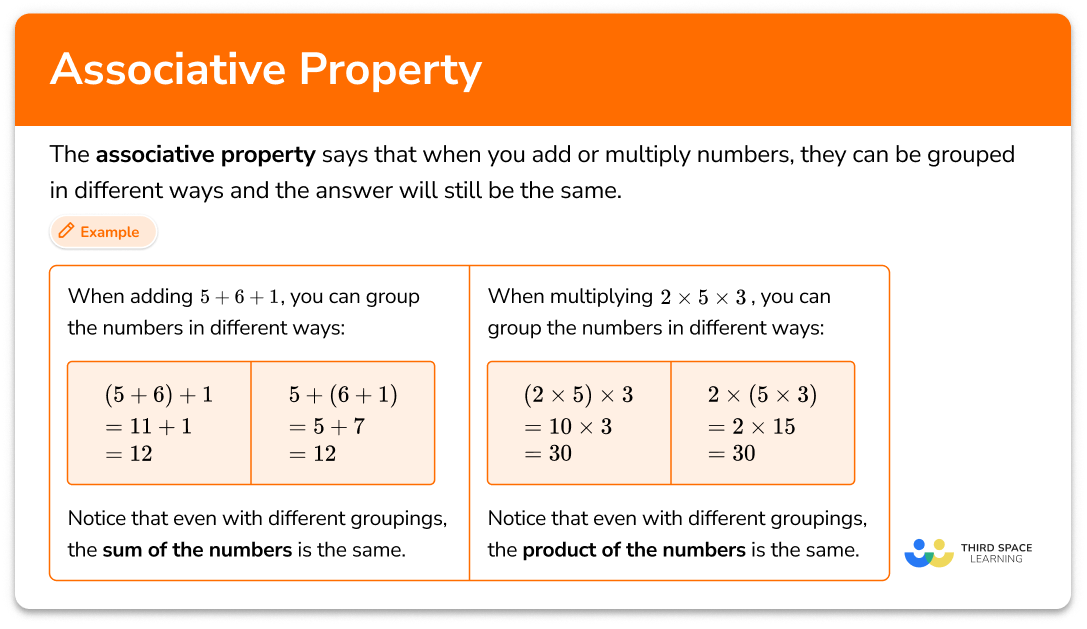Associative property

Associative property step-by-step topic guide, detailed examples, practice questions, teaching tips and FAQs.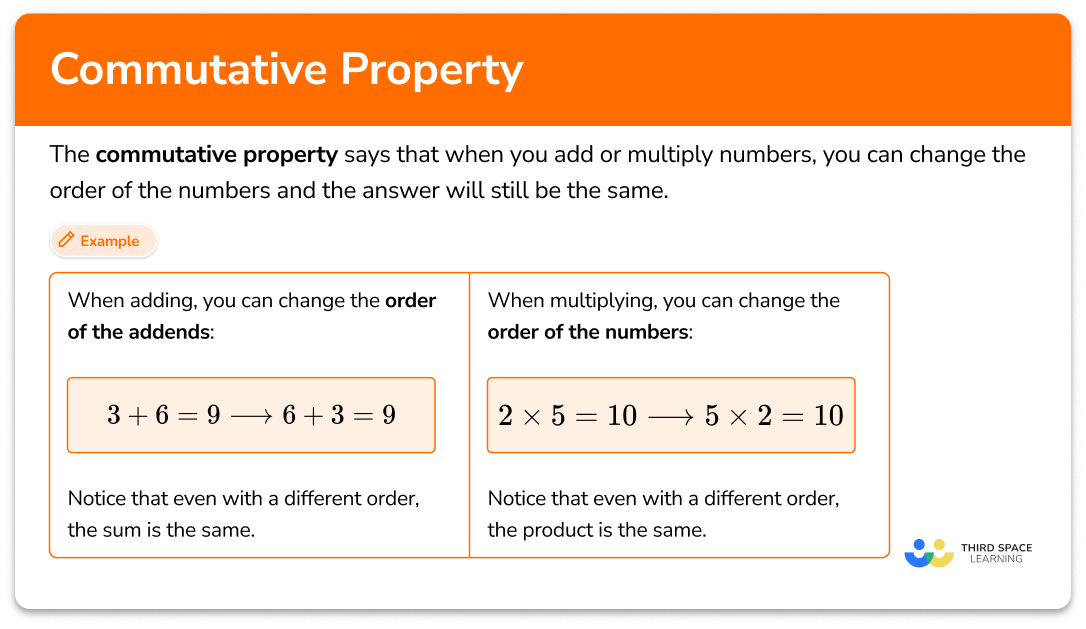Commutative property

Commutative property step-by-step topic guide, detailed examples, practice questions, teaching tips and FAQs.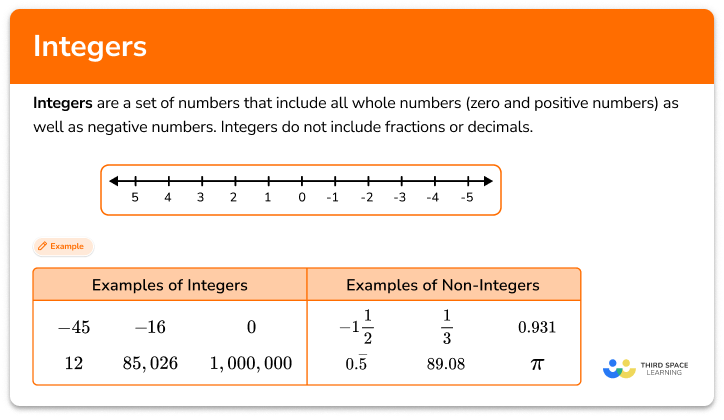Integers

Integers step-by-step topic guide, detailed examples, practice questions, teaching tips and FAQs.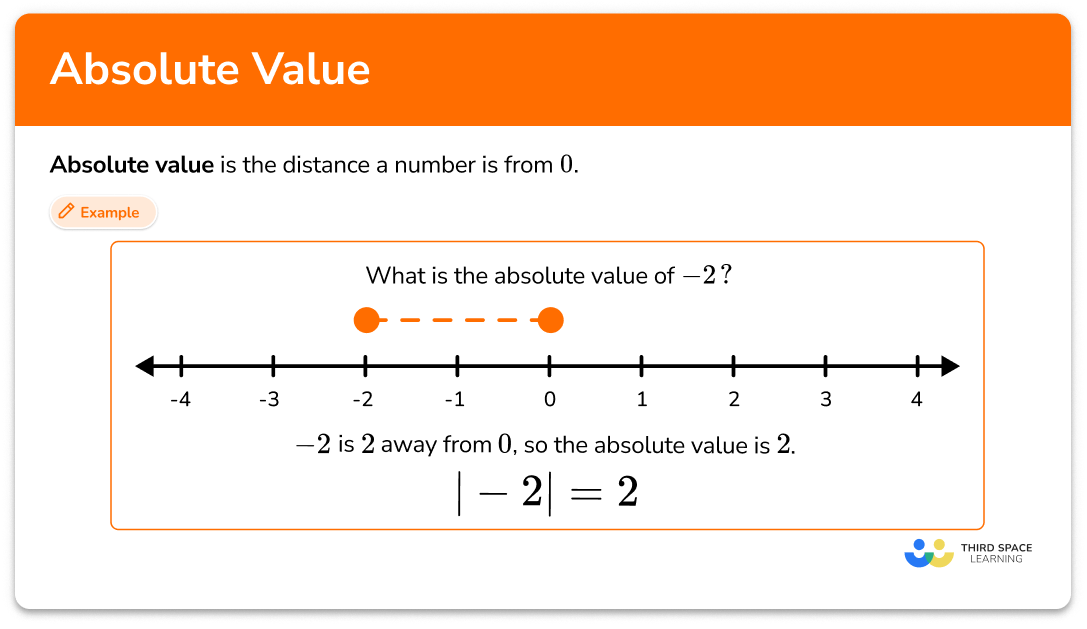Absolute value

Absolute value step-by-step topic guide, detailed examples, practice questions, teaching tips and FAQs.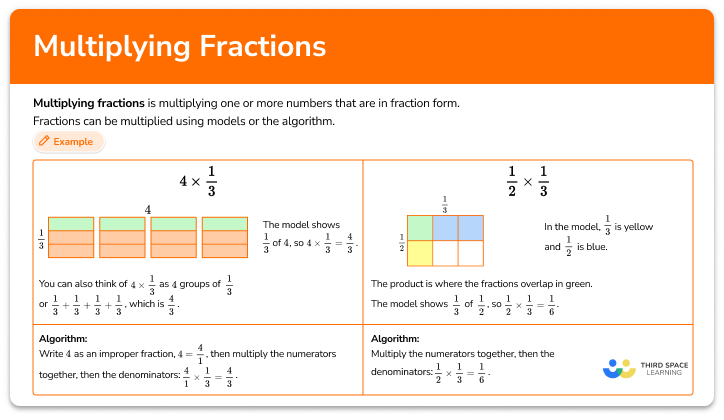Multiplying fractions

Multiplying fractions step-by-step topic guide, detailed examples, practice questions, teaching tips and FAQs.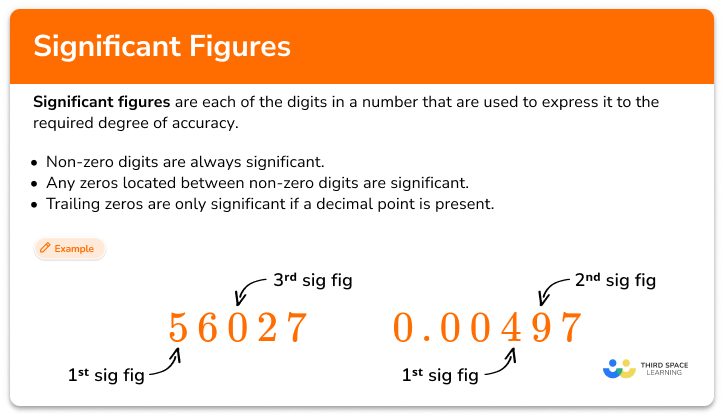Significant figures

Significant figures step-by-step topic guide, detailed examples, practice questions, teaching tips and FAQs.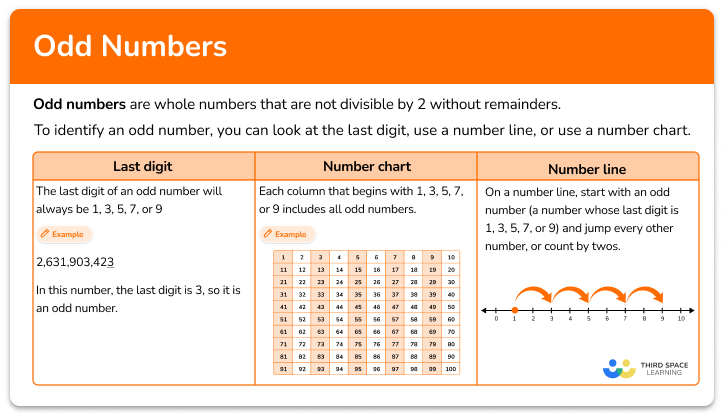Odd numbers

Odd numbers step-by-step topic guide, detailed examples, practice questions, teaching tips and FAQs.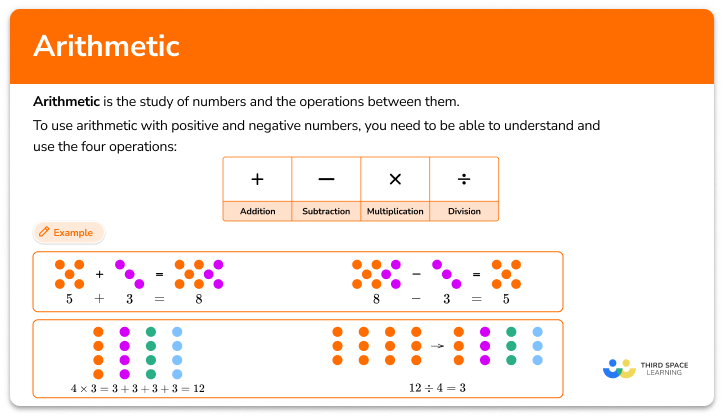Arithmetic

Arithmetic step-by-step topic guide, detailed examples, practice questions, teaching tips and FAQs.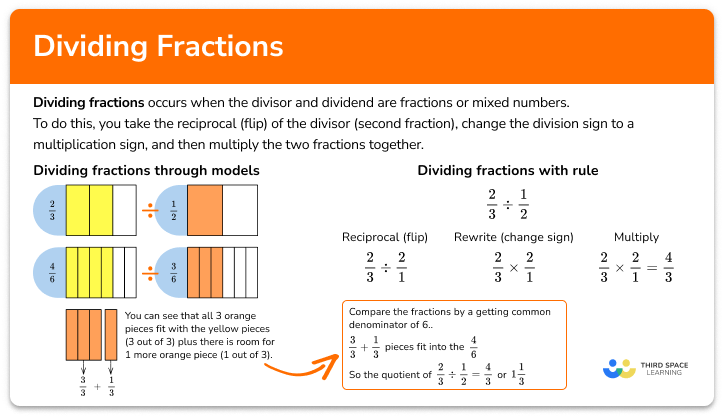Dividing fractions

Dividing fractions step-by-step topic guide, detailed examples, practice questions, teaching tips and FAQs.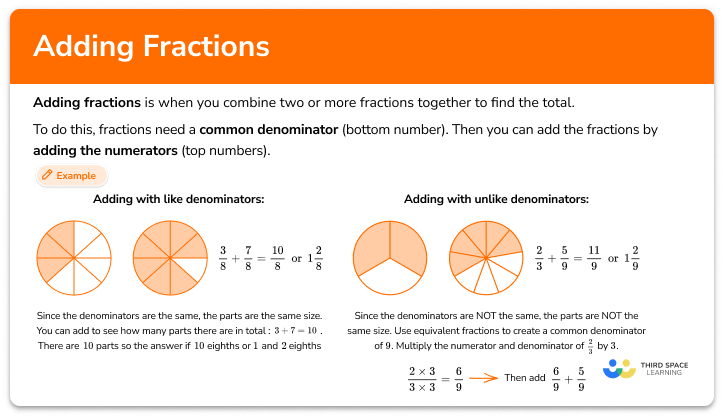Adding fractions step-by-step topic guide, detailed examples, practice questions, teaching tips and FAQs.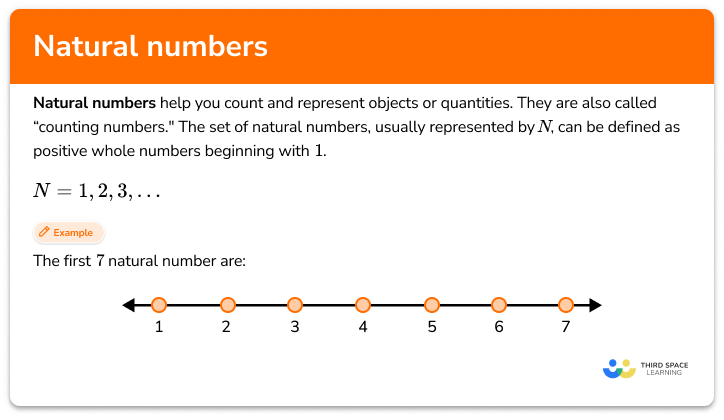Natural numbers

Natural numbers step-by-step topic guide, detailed examples, practice questions, teaching tips and FAQs.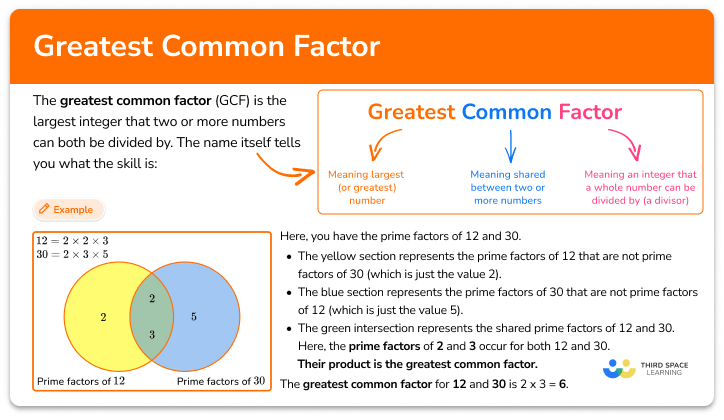Greatest common factor

Greatest common factor step-by-step topic guide, detailed examples, practice questions, teaching tips and FAQs.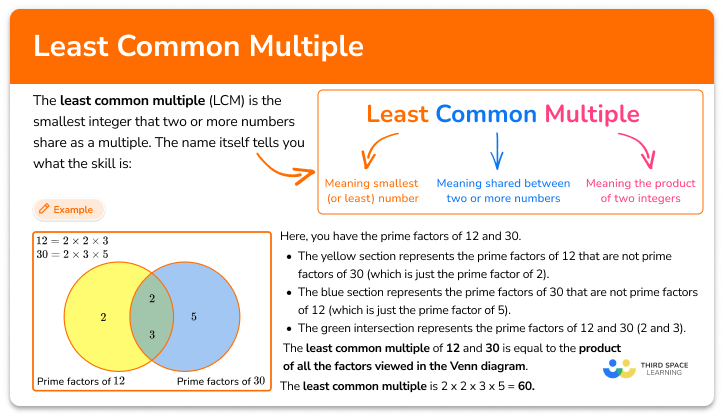Least common multiple

Least common multiple step-by-step topic guide, detailed examples, practice questions, teaching tips and FAQs.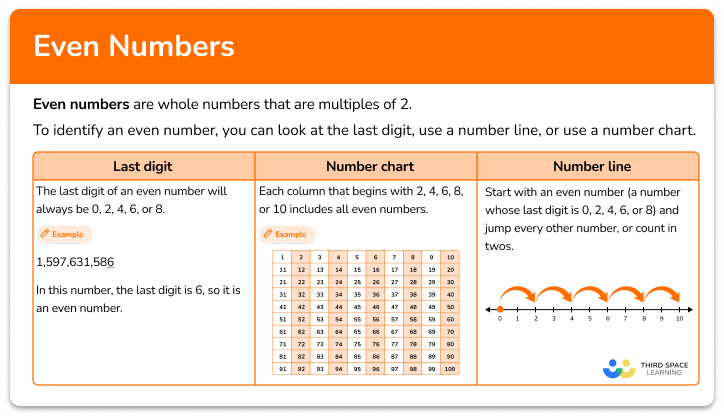Even numbers

Even numbers step-by-step topic guide, detailed examples, practice questions, teaching tips and FAQs.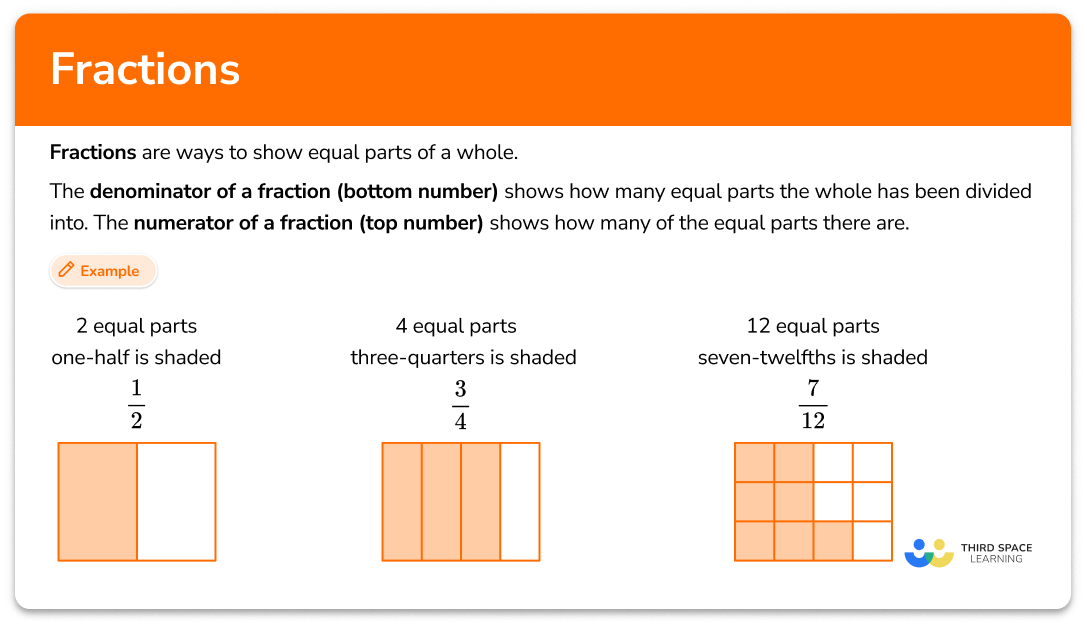Fractions

Fractions step-by-step topic guide, detailed examples, practice questions, teaching tips and FAQs.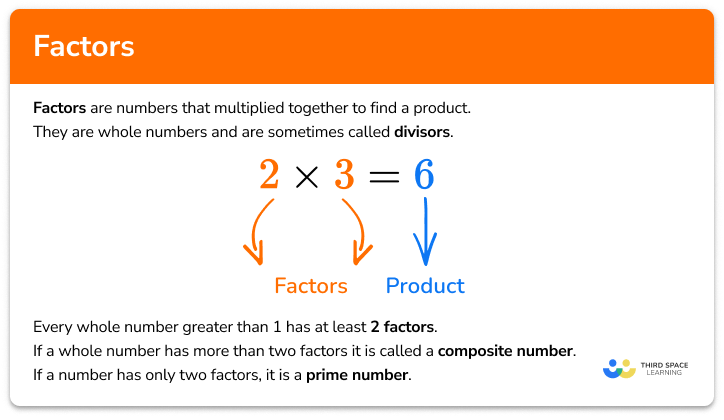Factors

Factors step-by-step topic guide, detailed examples, practice questions, teaching tips and FAQs.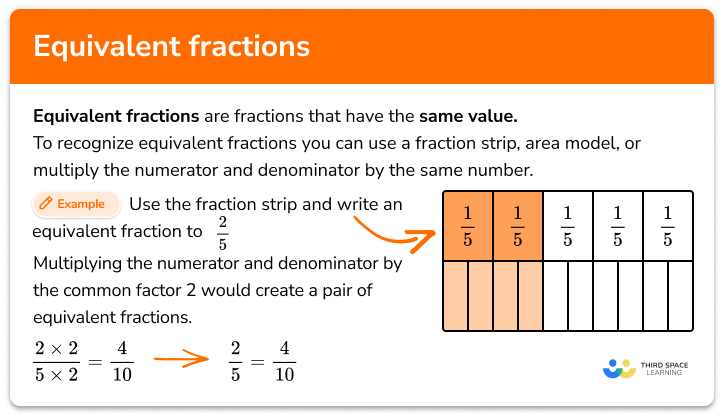Equivalent fractions

Equivalent fractions step-by-step topic guide, detailed examples, practice questions, teaching tips and FAQs.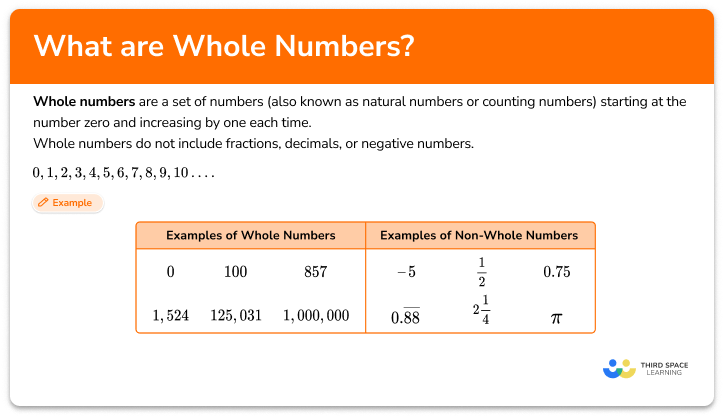Whole numbers

Whole numbers step-by-step topic guide, detailed examples, practice questions, teaching tips and FAQs.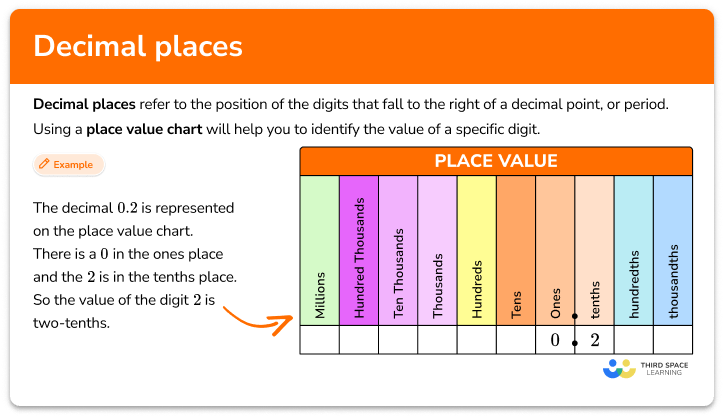Decimal places

Decimal places step-by-step topic guide, detailed examples, practice questions, teaching tips and FAQs.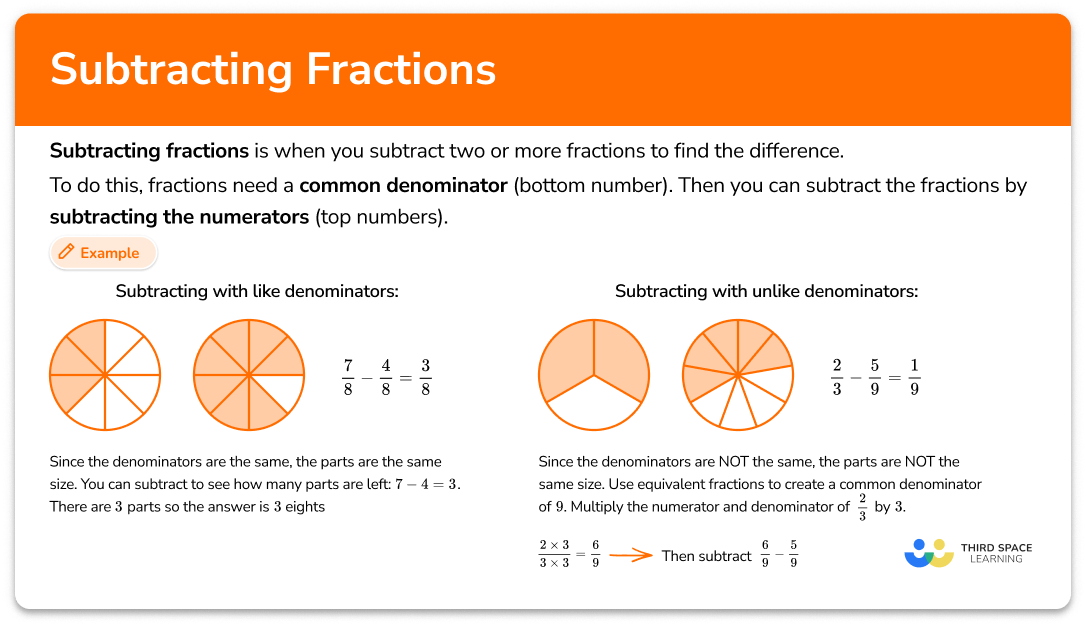Subtracting fractions

Subtracting fractions step-by-step topic guide, detailed examples, practice questions, teaching tips and FAQs.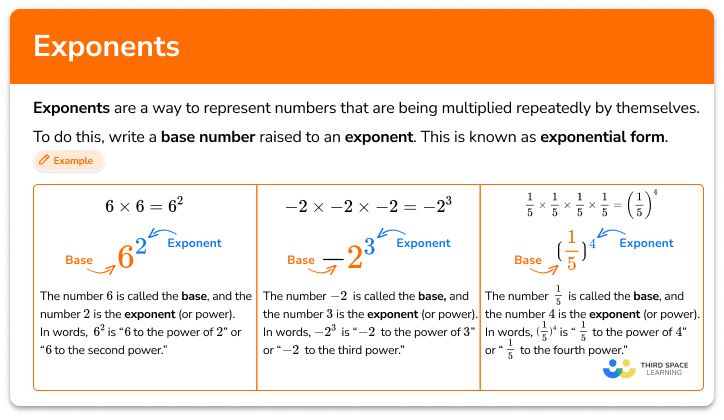Exponents

Exponents step-by-step topic guide, detailed examples, practice questions, teaching tips and FAQs.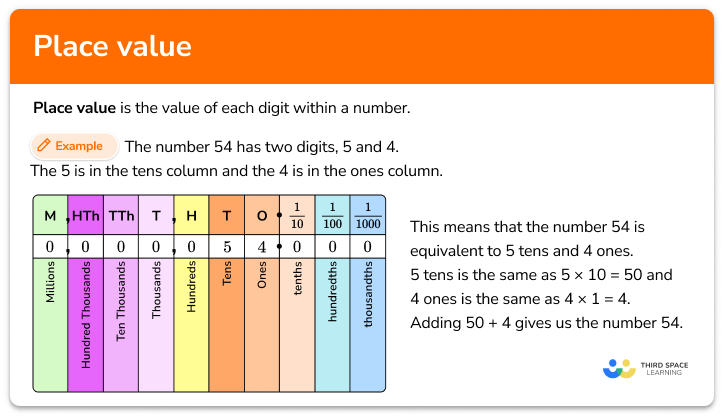Place value

Place value step-by-step topic guide, detailed examples, practice questions, teaching tips and FAQs.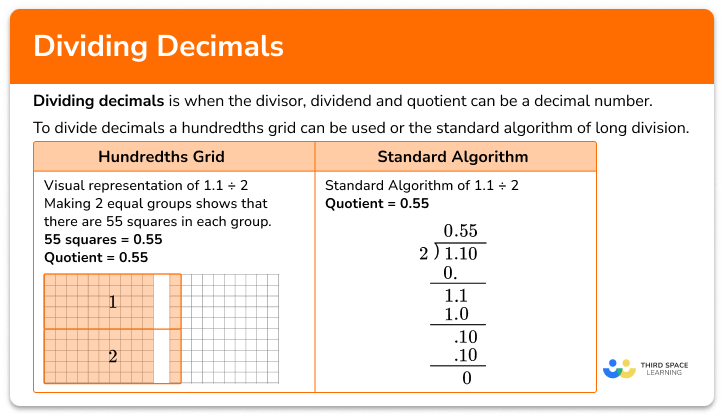Dividing decimals

Dividing decimals step-by-step topic guide, detailed examples, practice questions, teaching tips and FAQs.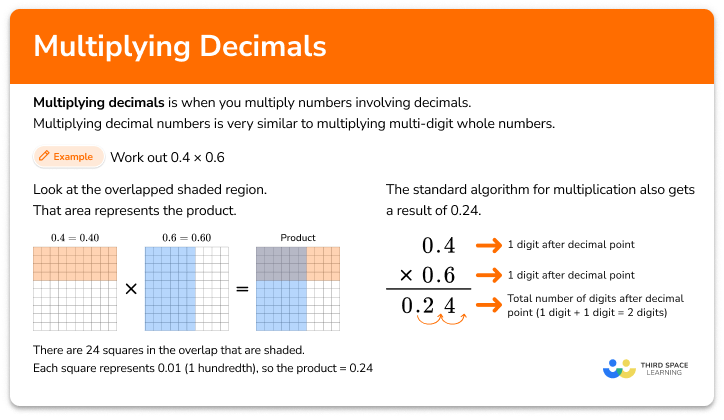Multiplying decimals

Multiplying decimals step-by-step topic guide, detailed examples, practice questions, teaching tips and FAQs.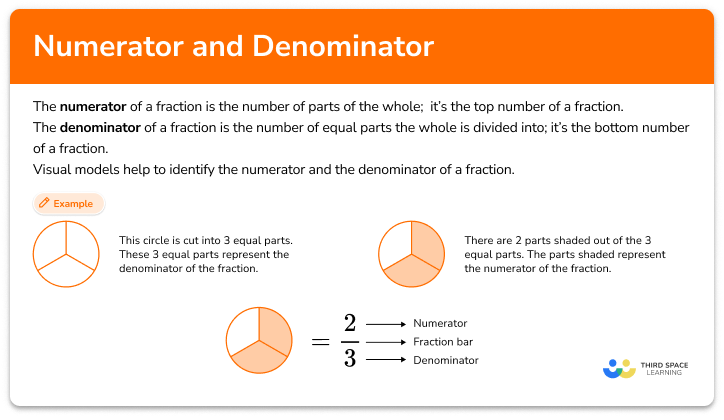Numerator and denominator

Numerator and denominator step-by-step topic guide, detailed examples, practice questions, teaching tips and FAQs.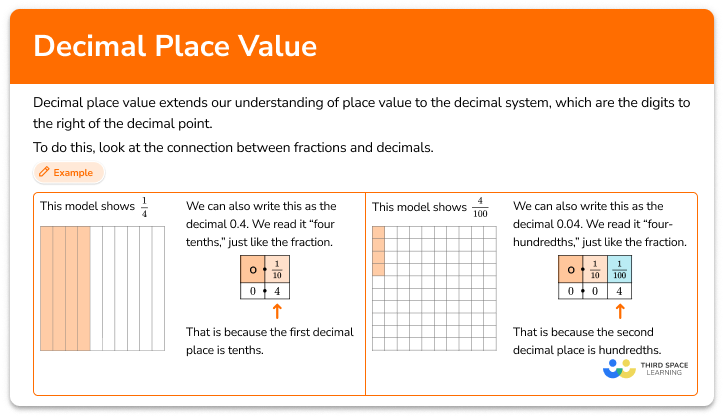Decimal place value

Decimal place value step-by-step topic guide, detailed examples, practice questions, teaching tips and FAQs.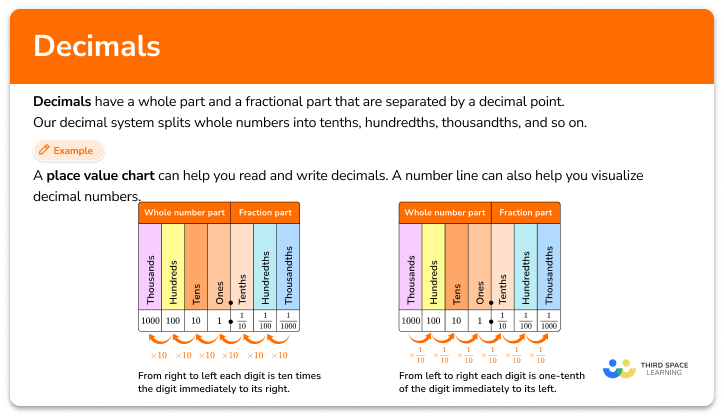Decimals

Decimals step-by-step topic guide, detailed examples, practice questions, teaching tips and FAQs.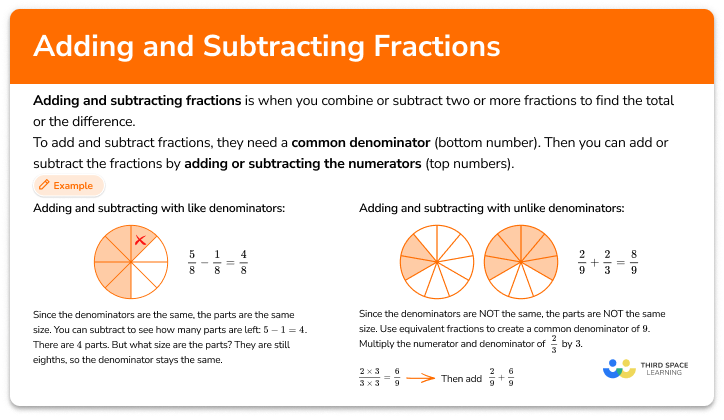Adding and subtracting fractions step-by-step topic guide, detailed examples, practice questions, teaching tips and FAQs.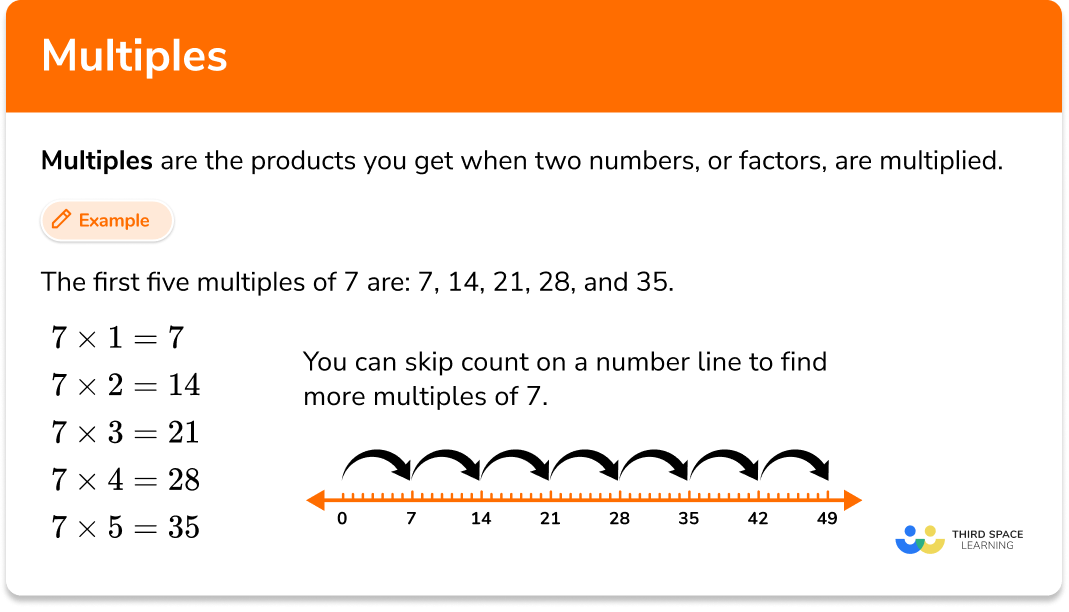Multiples

Multiples step-by-step topic guide, detailed examples, practice questions, teaching tips and FAQs.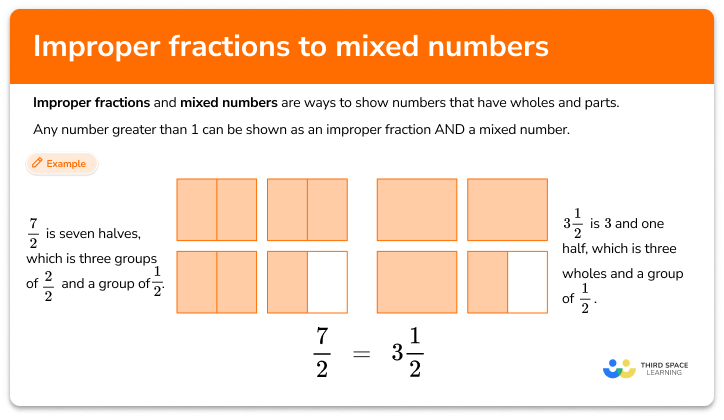Improper fraction to mixed number

Improper fraction to mixed number step-by-step topic guide, detailed examples, practice questions, teaching tips and FAQs.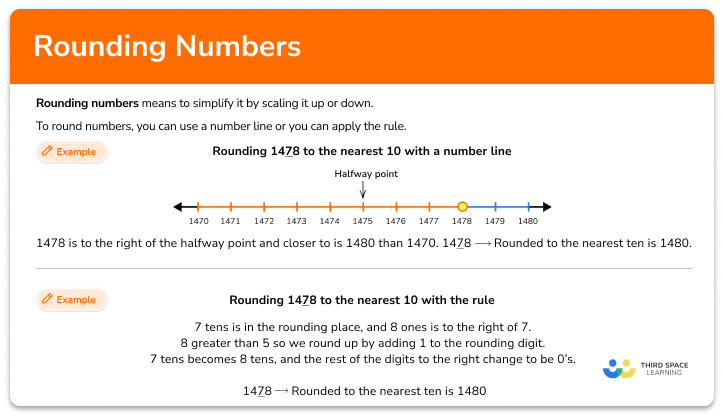Rounding numbers

Rounding numbers step-by-step topic guide, detailed examples, practice questions, teaching tips and FAQs.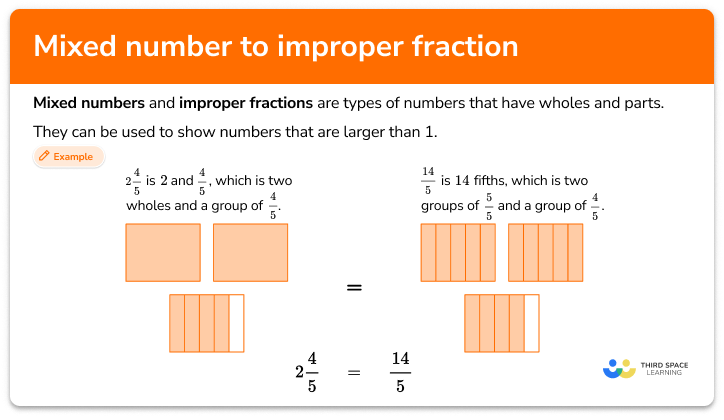Mixed number to improper fraction

Mixed number to improper fraction step-by-step topic guide, detailed examples, practice questions, teaching tips and FAQs.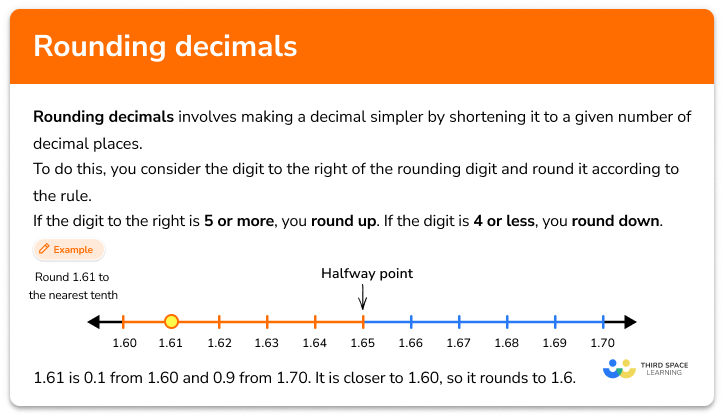Rounding decimals

Rounding decimals step-by-step topic guide, detailed examples, practice questions, teaching tips and FAQs.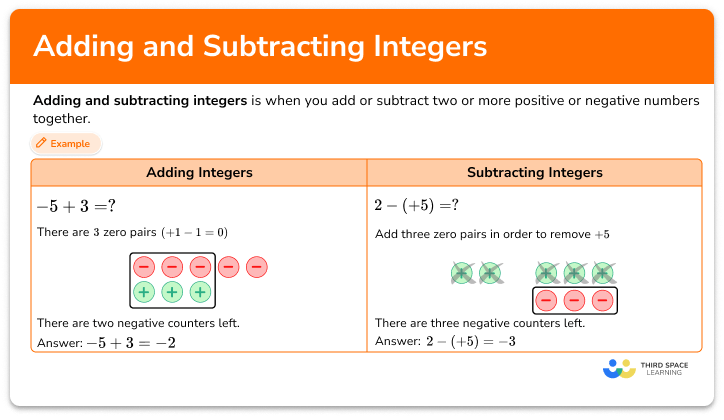Adding and subtracting integers step-by-step topic guide, detailed examples, practice questions, teaching tips and FAQs.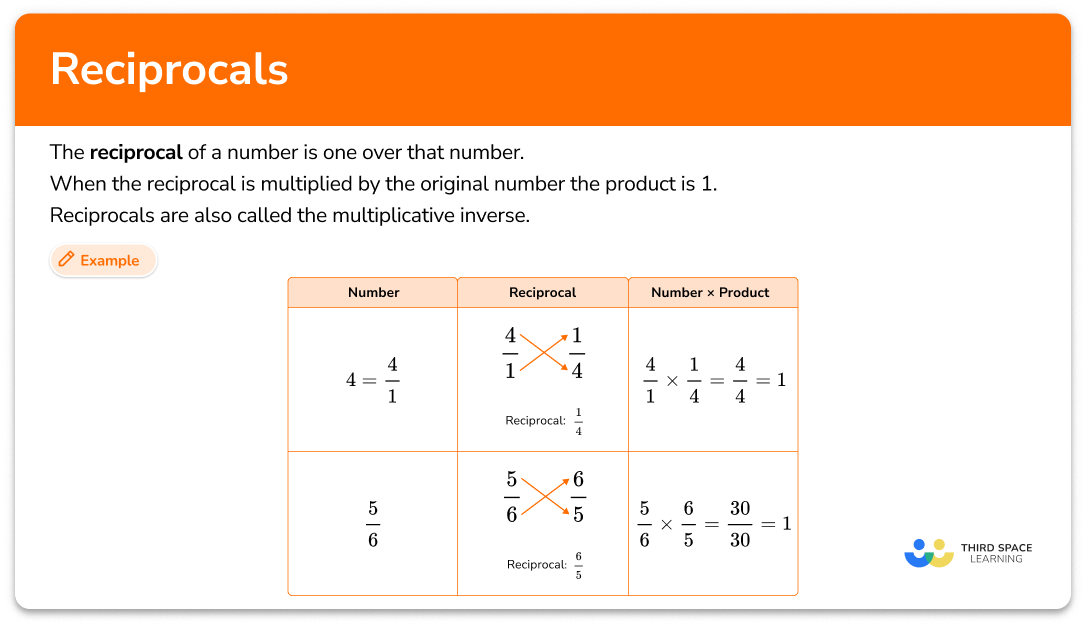Reciprocal math

Reciprocal math step-by-step topic guide, detailed examples, practice questions, teaching tips and FAQs.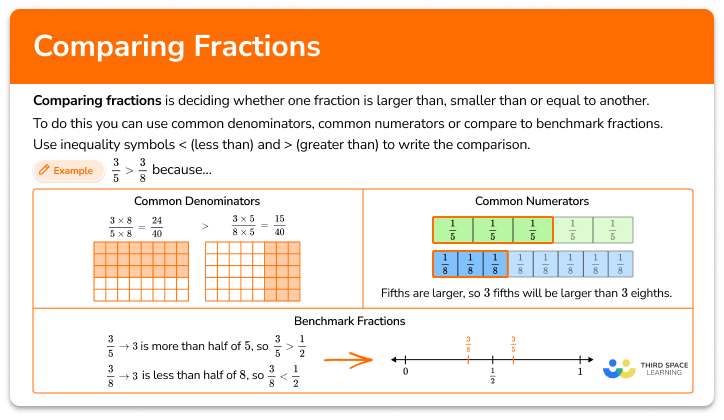Comparing fractions

Comparing fractions step-by-step topic guide, detailed examples, practice questions, teaching tips and FAQs.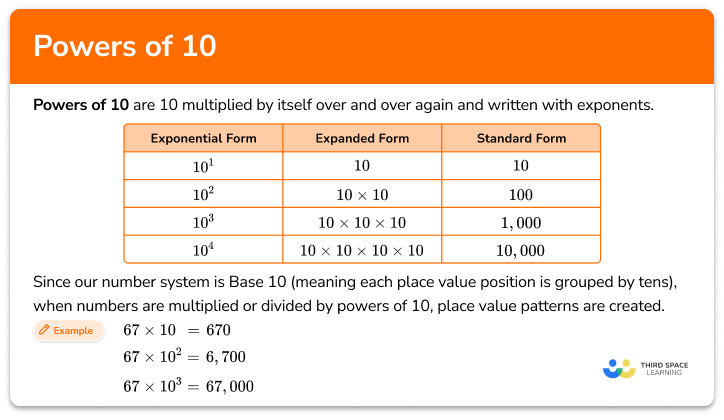Powers of 10

Powers of 10 step-by-step topic guide, detailed examples, practice questions, teaching tips and FAQs.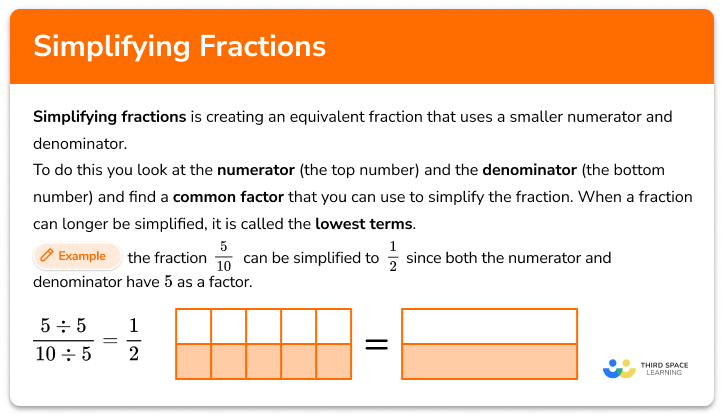Simplifying fractions

Simplifying fractions step-by-step topic guide, detailed examples, practice questions, teaching tips and FAQs.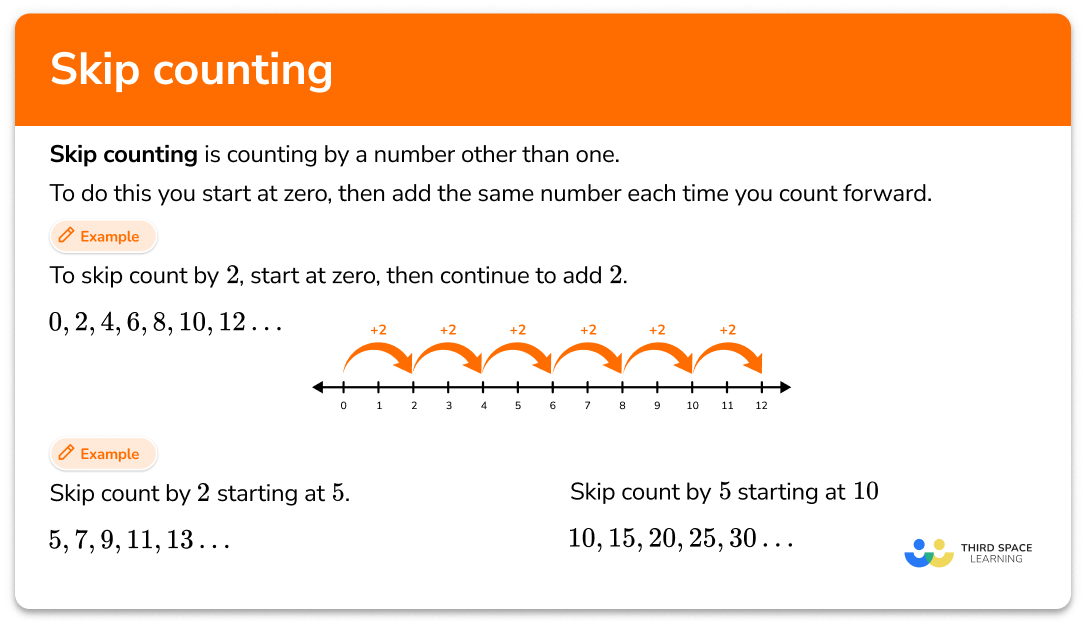Skip counting

Skip counting step-by-step topic guide, detailed examples, practice questions, teaching tips and FAQs.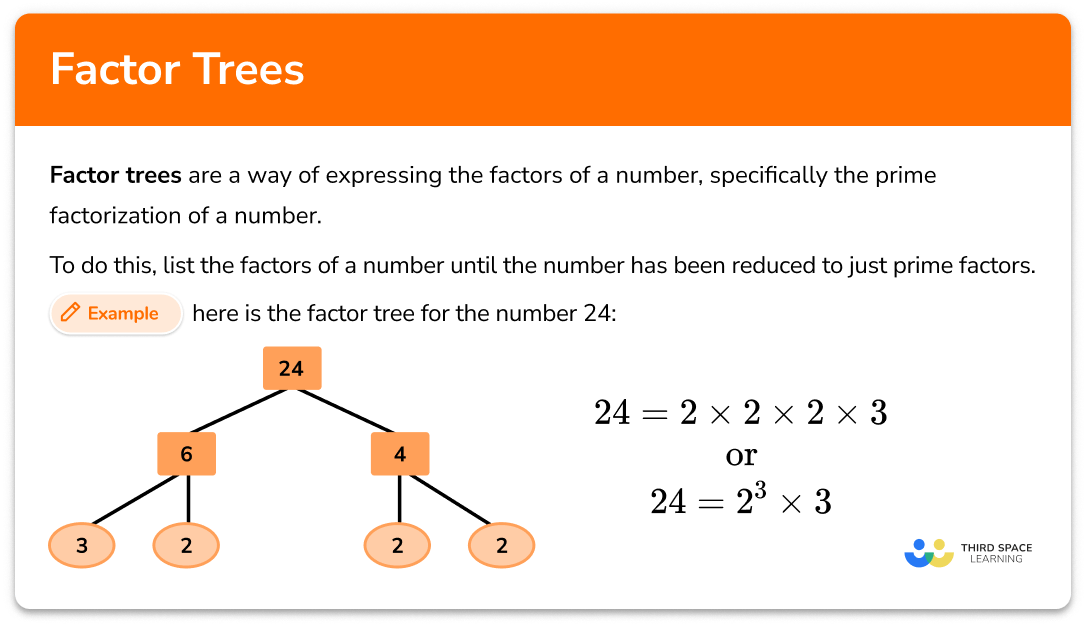Factor tree

Factor trees step-by-step topic guide, detailed examples, practice questions, teaching tips and FAQs.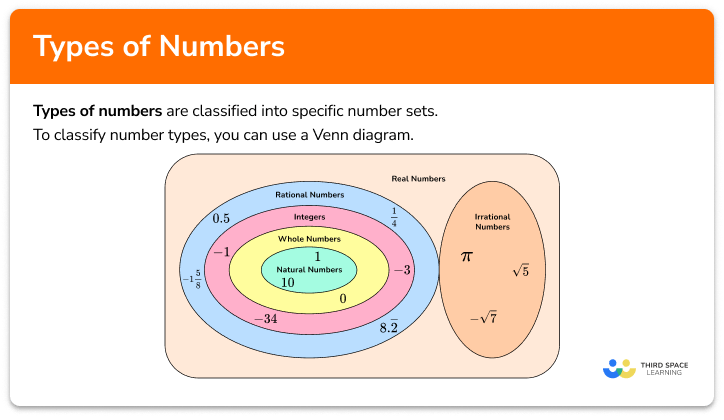Types of numbers

Types of numbers step-by-step topic guide, detailed examples, practice questions, teaching tips and FAQs.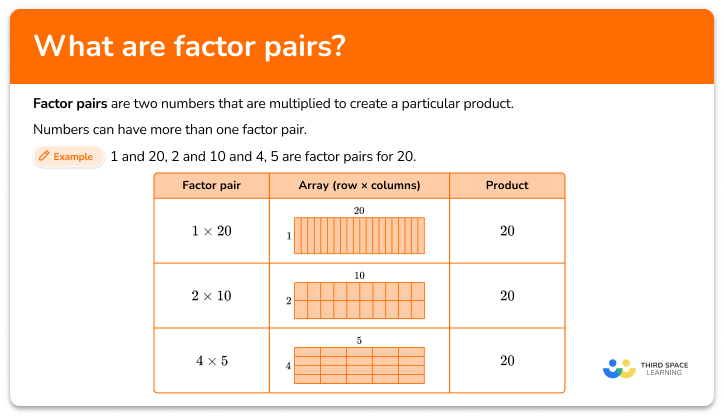Factor pairs

Factor pairs step-by-step topic guide, detailed examples, practice questions, teaching tips and FAQs.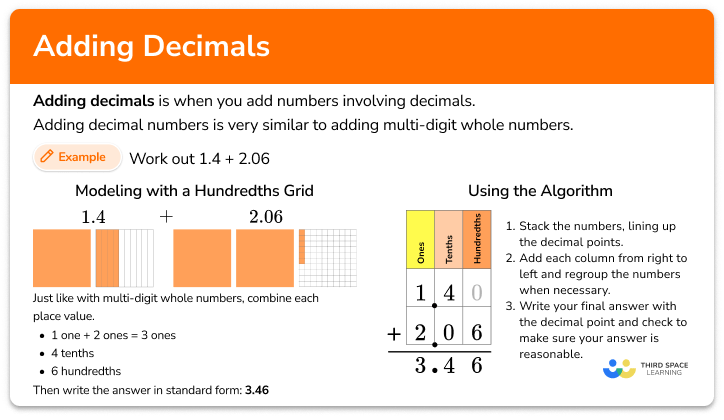Adding decimals step-by-step topic guide, detailed examples, practice questions, teaching tips and FAQs.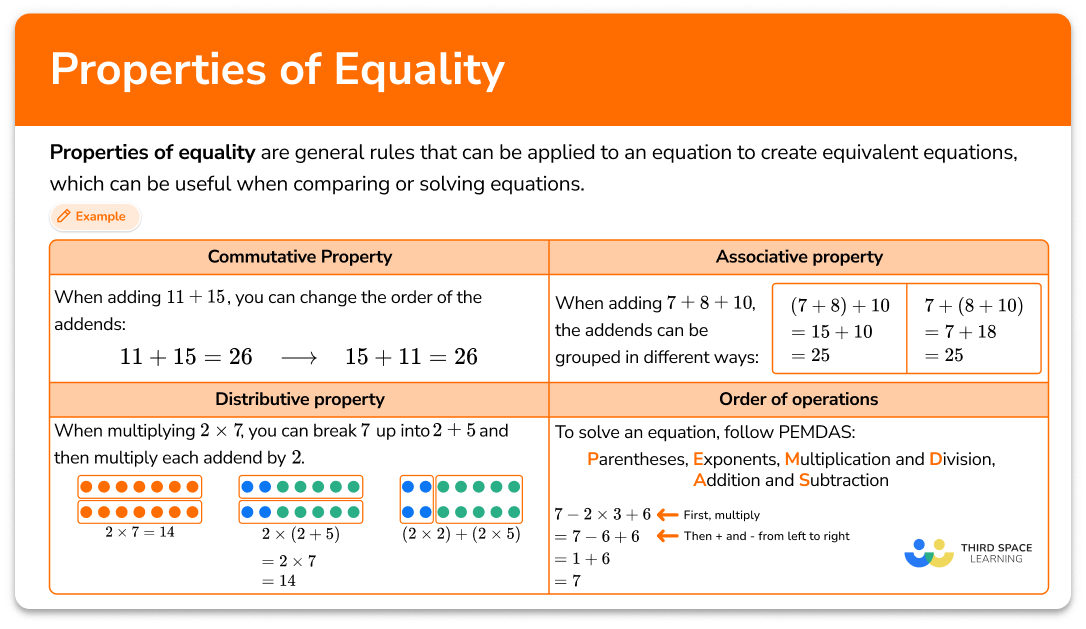Properties of equality

Properties of equality step-by-step topic guide, detailed examples, practice questions, teaching tips and FAQs.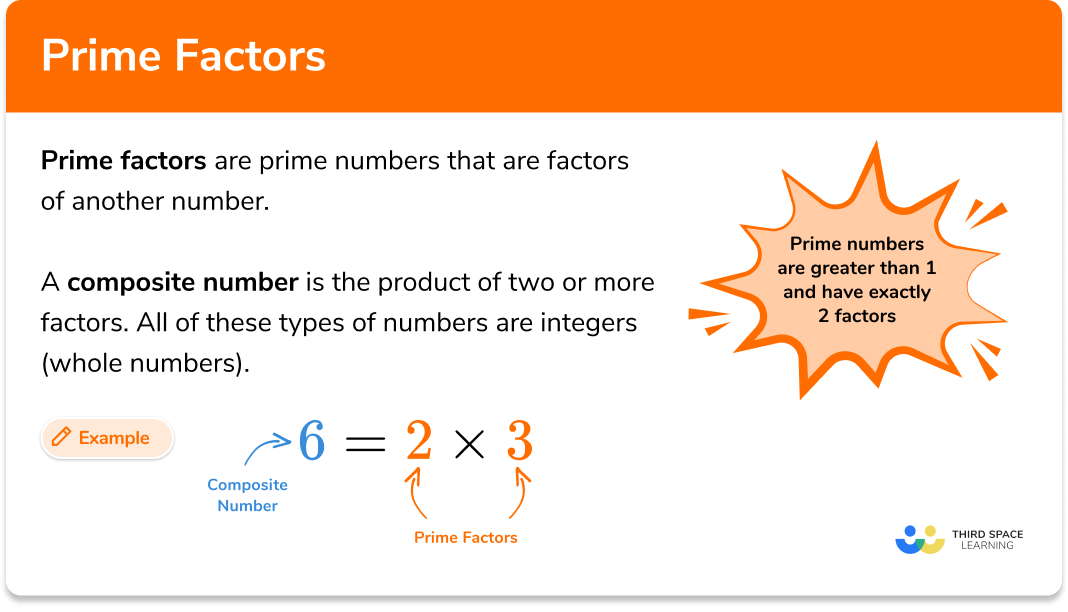Prime factors

Prime factors step-by-step topic guide, detailed examples, practice questions, teaching tips and FAQs.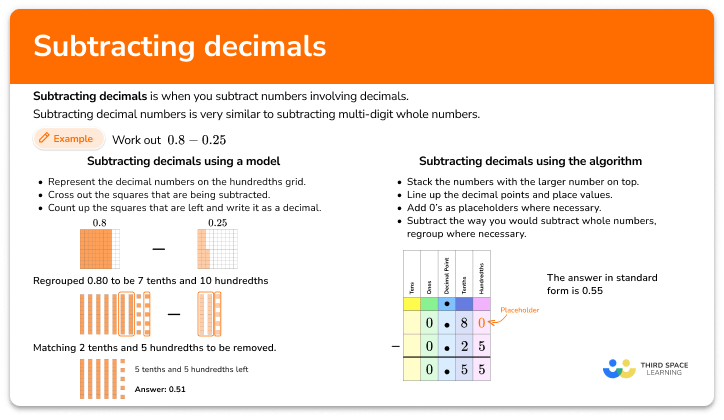Subtracting decimals

Subtracting decimals step-by-step topic guide, detailed examples, practice questions, teaching tips and FAQs.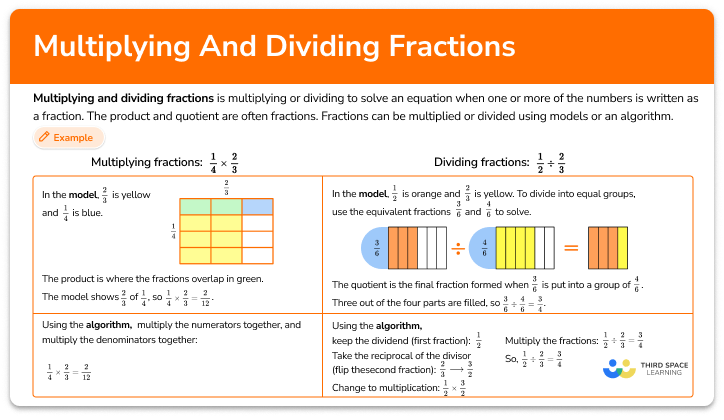Multiplying and dividing fractions

Multiplying and dividing fractions step-by-step topic guide, detailed examples, practice questions, teaching tips and FAQs.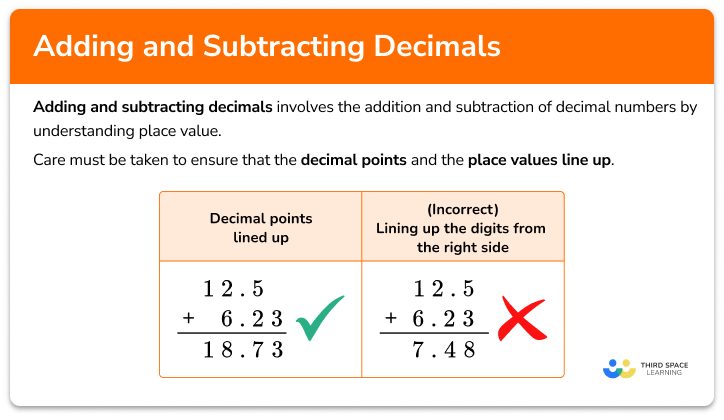Adding and subtracting decimals step-by-step topic guide, detailed examples, practice questions, teaching tips and FAQs.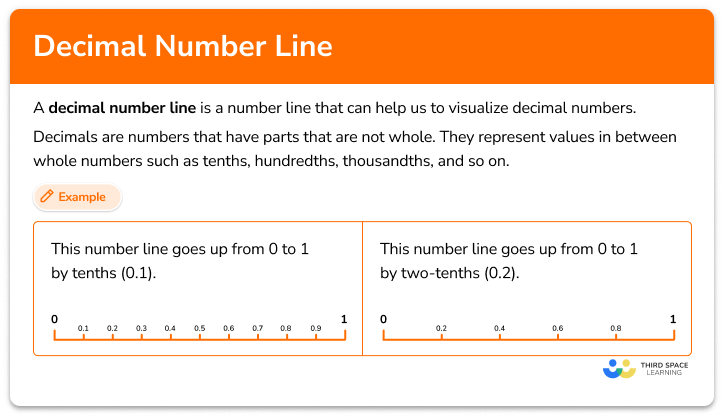Decimal number line

Decimal number line step-by-step topic guide, detailed examples, practice questions, teaching tips and FAQs.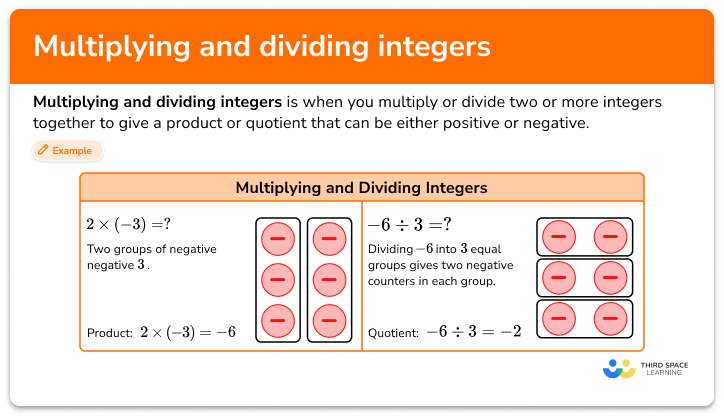Multiplying and dividing integers

Multiplying and dividing integers step-by-step topic guide, detailed examples, practice questions, teaching tips and FAQs.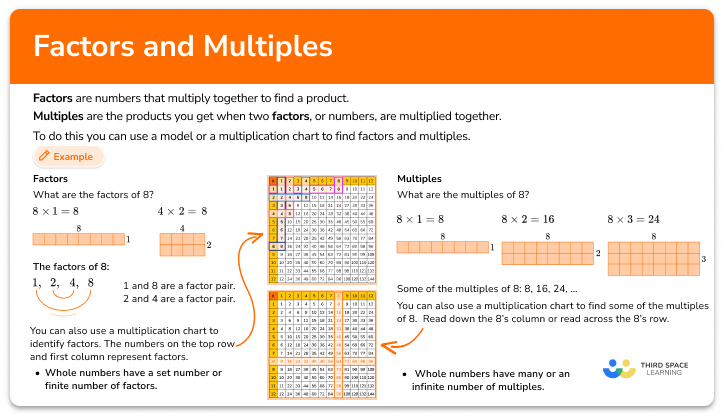Factors and multiples

Factors and multiples step-by-step topic guide, detailed examples, practice questions, teaching tips and FAQs.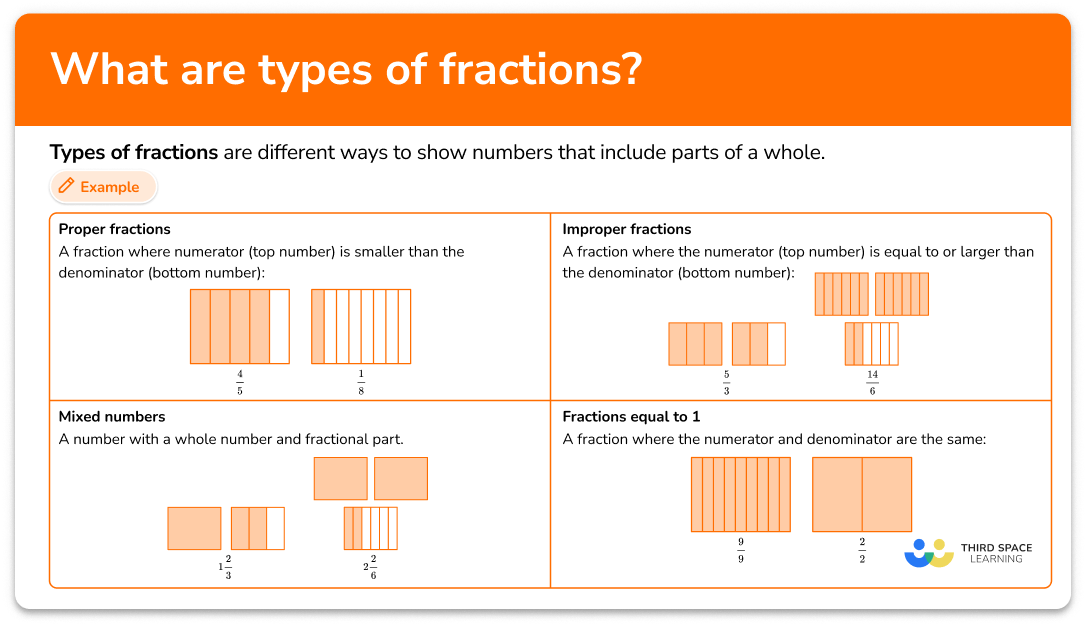Types of fractions

Types of fractions step-by-step topic guide, detailed examples, practice questions, teaching tips and FAQs.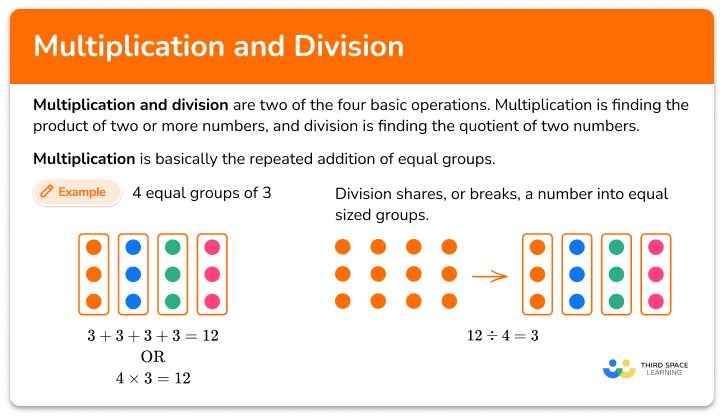Multiplication and division

Multiplication and division step-by-step topic guide, detailed examples, practice questions, teaching tips and FAQs.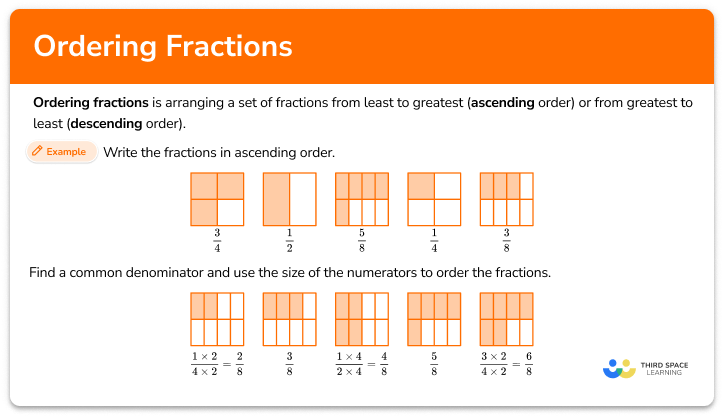Ordering fractions

Ordering fractions step-by-step topic guide, detailed examples, practice questions, teaching tips and FAQs.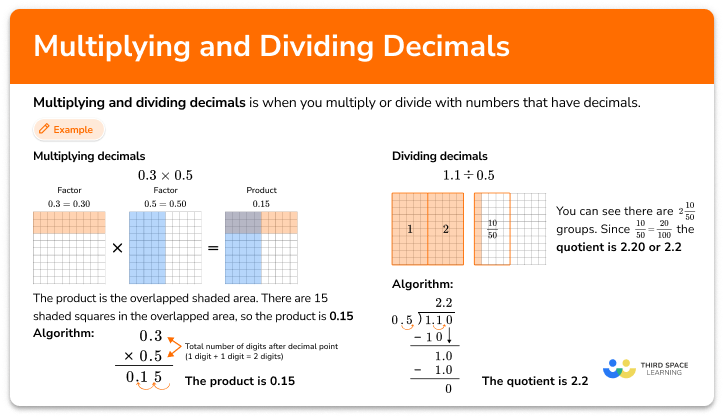Multiplying and dividing decimals

Multiplying and dividing decimals step-by-step topic guide, detailed examples, practice questions, teaching tips and FAQs.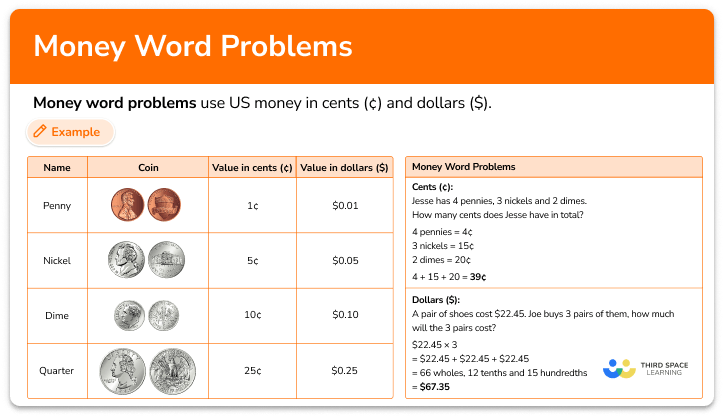Money word problems

Money word problems step-by-step topic guide, detailed examples, practice questions, teaching tips and FAQs.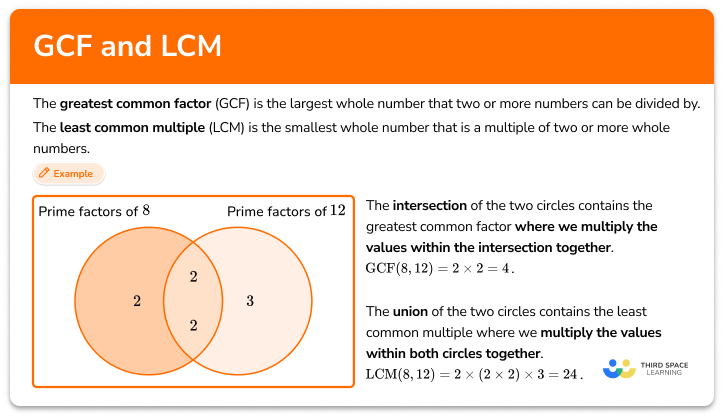GCF and LCM

GCF and LCM step-by-step topic guide, detailed examples, practice questions, teaching tips and FAQs.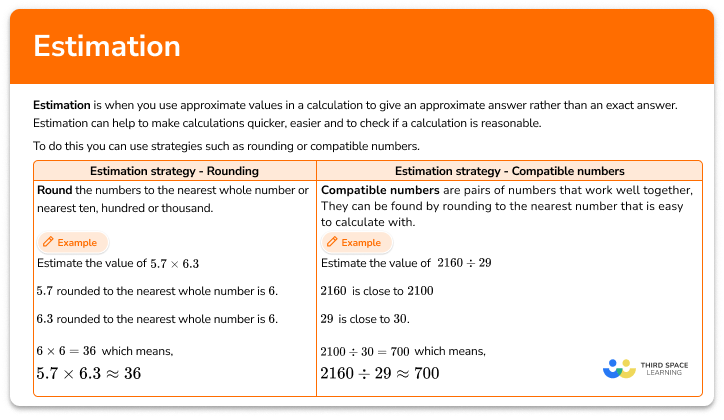Estimation

Estimation step-by-step topic guide, detailed examples, practice questions, teaching tips and FAQs.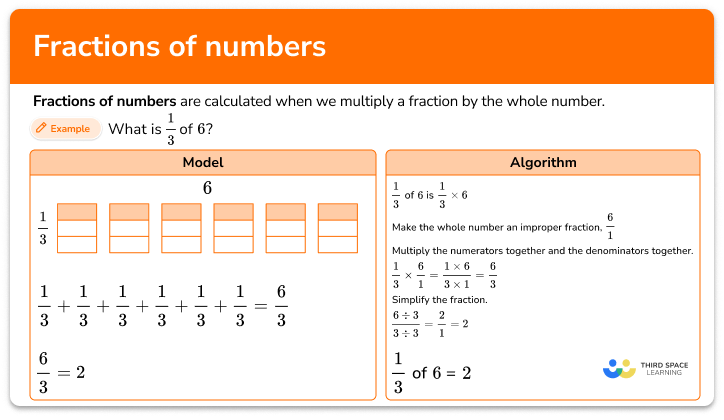Fractions of numbers

Fractions of numbers step-by-step topic guide, detailed examples, practice questions, teaching tips and FAQs.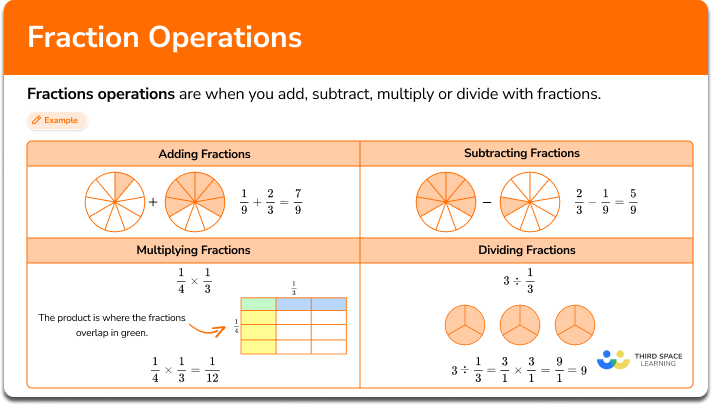Fractions operations

Fractions operations step-by-step topic guide, detailed examples, practice questions, teaching tips and FAQs.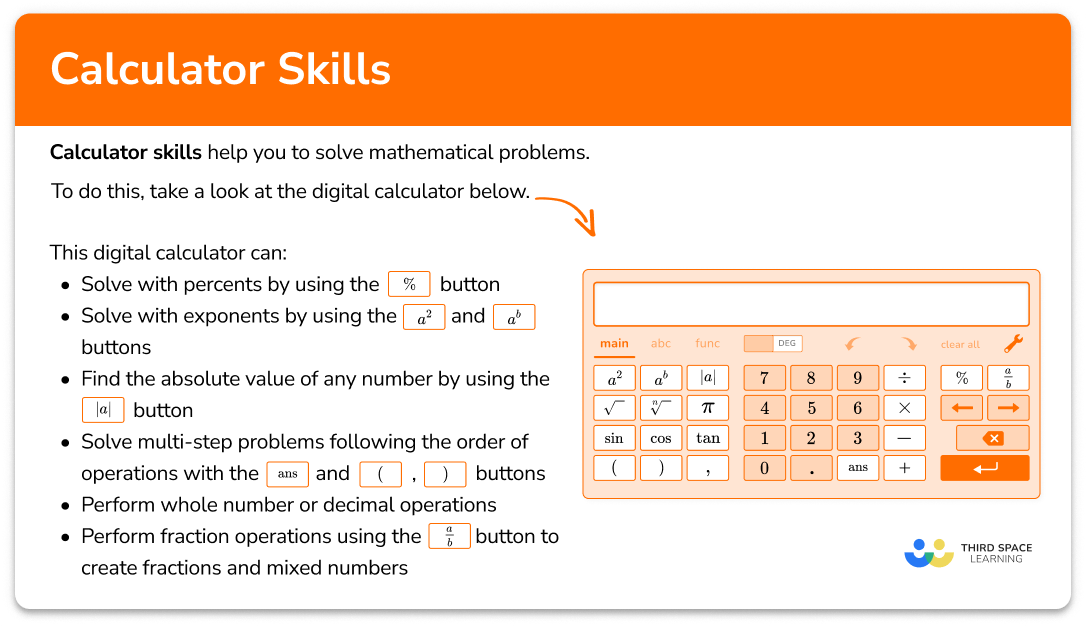Calculator skills

Calculator skills step-by-step topic guide, detailed examples, practice questions, teaching tips and FAQs.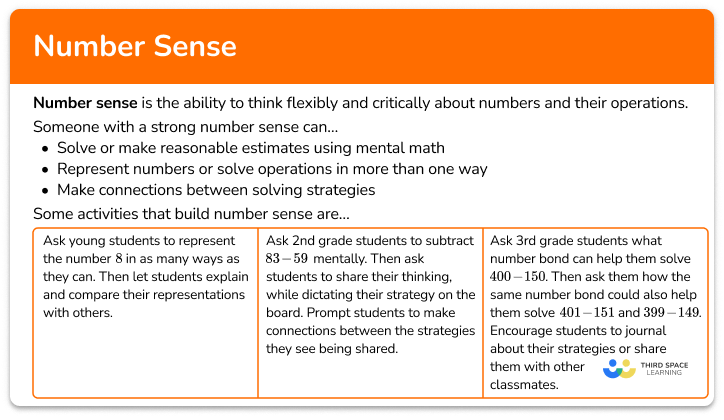Number sense

Number sense step-by-step topic guide, detailed examples, practice questions, teaching tips and FAQs.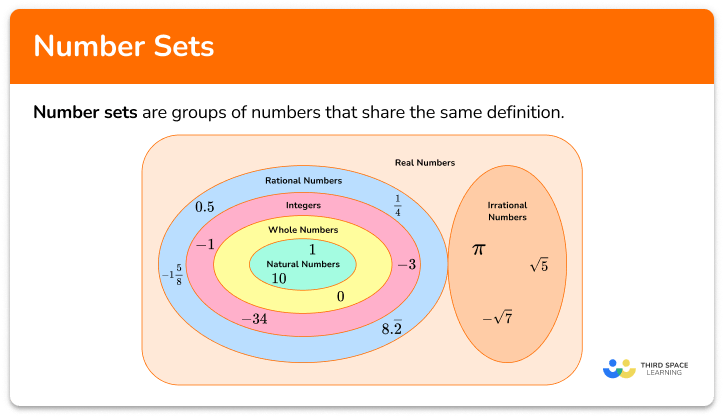Number sets

Number sets step-by-step topic guide, detailed examples, practice questions, teaching tips and FAQs.